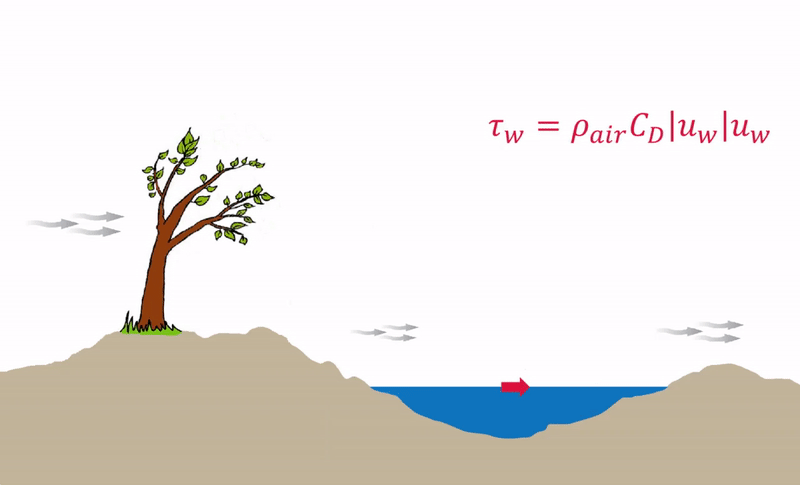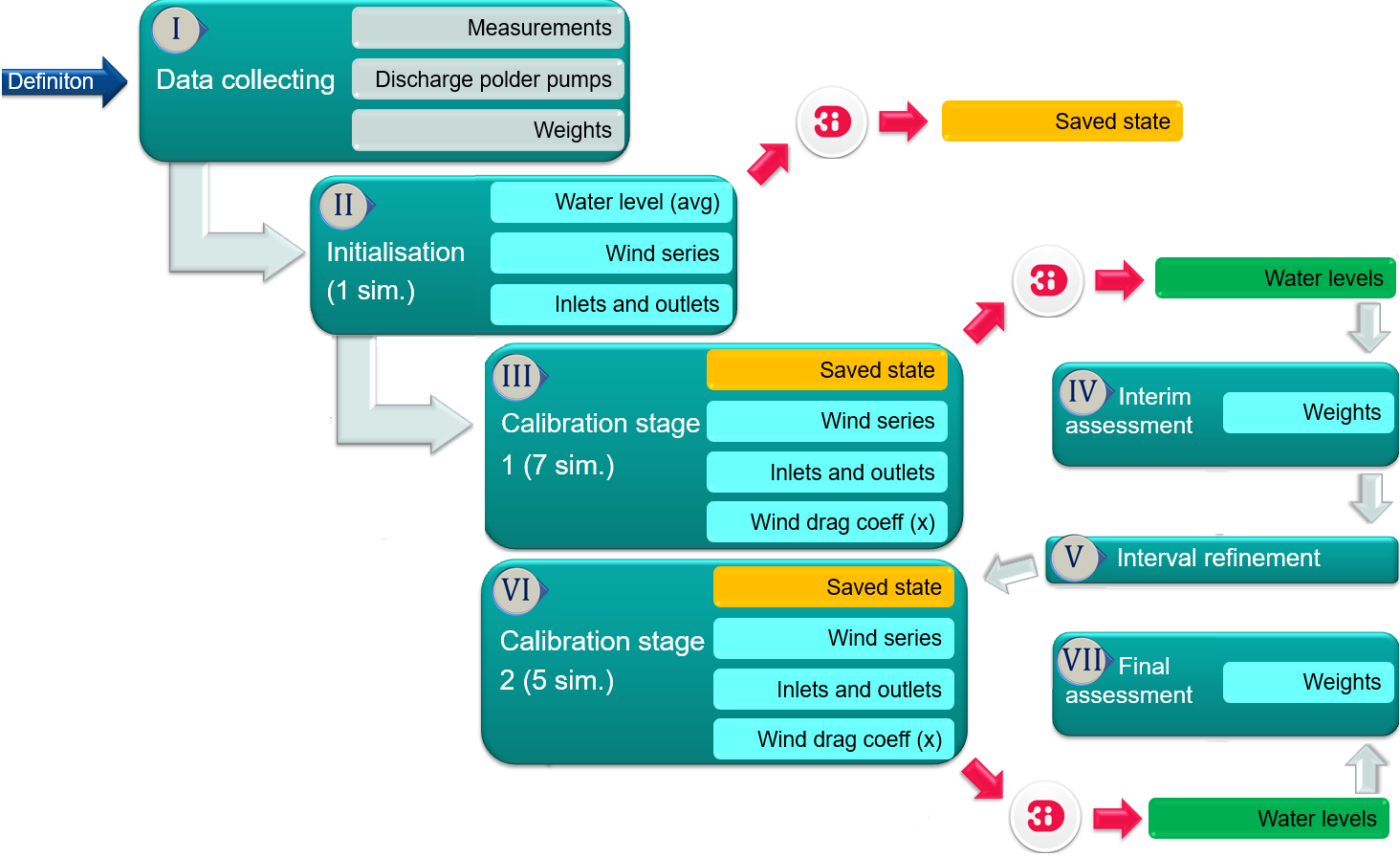# Calibrate (wind set-up) using 3Di

With 3Di we have semi-automatically calibrated the wind drag coefficient using API and scripting.

Calibrating a hydrodynamic model requires a lot of work. By smart use of 3Di’s API this becomes a lot more feasible. In this use case we look at the momentum transfer of wind to waterbodies. In 3Di models, this effect is scaled with the wind drag coefficient. Existing theory suggests that this coefficient can vary a lot within the same water system and for different wind directions.Using model calibration, we have found optimal wind drag coefficients for different historic wind events on different locations. The workflow is depicted in the following figure:Firstly, an initial calculation is performed. The outcome of this calculation delivers a so-called saved stated, which will be used as a starting point for the following model runs.

In the first calibration phase, seven simulations were run with a different wind drag coefficient. The results were analysed and after a refinement a second calibration phase was performed with another five calculations. As the wind drag coefficient can vary per location in the water system (e.g., a large lake or a smaller canal), this calculation has been done twelve times. In total this amounts to several hunderds of calculations.

This sounds like a lot of work, but by using 3Di this can be done automatically. The calculations are done in the cloud, only the results were downloaded for analyses. Using a python script, the results were downloaded and automatically assessed using an objective function that compares simulated water levels with measured water levels. Using this method, finding the optimal wind drag coefficient per location equals to pressing just a single button!

The study demonstrated relations between the location in the water system, the wind direction and the optimal wind drag coefficient. Overall, the drag coefficient was significantly lower at areas with smaller fetches, due to the geometry of the water bodies in-line with the wind direction. Less momentum transmission between the wind and the water occurred here, as wind shielding had a greater impact. Using these results, it is estimated how much the model accuracy can be improved with a varying wind drag coefficient (both in space and per wind direction), compared to the current 3Di model with a constant wind drag coefficient. The overall improvement of the model prediction for a spatially and wind direction varying wind drag coefficient is estimated at 44%.

Follow-up research where the model is improved with the wind drag coefficients resulting from this research can show the exact improvements.

An example of the model improvement is given in the figure below. The dark blue line resembles the simulated water levels of the reference model at some location in the water system, whereas the yellow line resembles the model with the optimal wind drag coefficient. The light blue line displays the measured water level. Notice how much the model can be improved by choosing the right wind drag coefficient!